# Meter Stick Math Lab

Take a meter stick. Create a right angled triangle anywhere in the room where a right angle is present, using the meter stick as the hypotenuse.Measure the two angles, and both shorter sides. Recreate your triangle on the applet ‘Triangles that have hypotenuse 1’ below, to see how accurate your measurements are!

As we know, a triangle has three sides and three angles. On a right-angled triangle, it is given that one angle is 90°.

As long as we are given two sides on a right angled triangle, or one side and one angle, we can calculate the remaining angle(s) and sides using values from the ‘triangle with hypotenuse 1’.

# Triangles that have hypotenuse length 1

On this applet, the hypotenuse has been set to 1.

(a) What angle makes the red and green side equal in length?

(b) What angle makes the adjacent side (red) 0.5?

(c) What is the relationship between the lengths of the red side, the green side and the hypotenuse?

(d) What does a very large angle do to the red side?

(e) What does a very large angle do to the green side?

(f) When the angle is 20°, the green side is 0.342; the red side is 0.94. At what angle do they swap, that is, the red is 0.342 and the green is 0.94?

(g) What does Pythagoras’ Theorem say about the length of the red side, the green side and the hypotenuse? Try it out for any angle.

# Create right angled triangles using one angle and the hypotenuse

Use this applet to create triangles that have the following:

 angle hyp opp adj other angle (a) 30° 10 5 8.66 60° (b) 30° 5 (c) 48° 7 (d) 10 4 (e) 10 4 (f) 12° 1 (g) 5.3 5.3

# Sines, Cosines, Tangents

The green and red sides of the right-angled triangle hypotenuse 1 are available on any scientific calculator.

On the applet above (hypotenuse 1), change the angle to 24°.

On a scientific calculator, ensure the screen says ‘DEG’ for degree mode; and type in sin(24°); cos(24°) and tan(24°).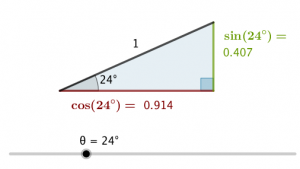It is possible that you notice that the green length; which is opposite the marked angle; is the sine of the marked angle (rounded to 2 d.p). The red, adjacent length is the cosine.

The tangent? That is the opposite side divided by the adjacent side (green over red). The tangent makes more sense when seen on a circle, as it is is a length marked on the tangent of the circle at the angle of reference.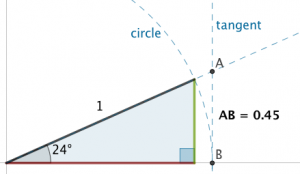Every other right-angled triangle is simply an enlargement of the right-angled triangle that has hypotenuse = 1.

Suppose we have a right-angled triangle with hypotenuse = 8 and given angle = 24°.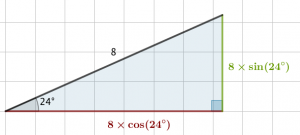Type inandon your scientific calculator. Verify your answers with the first applet above – change the hypotenuse to 8 and the angle to 24.

# In general

On a right angled triangle with hypotenuse length 1, the sine of an angle is the length of the side opposite that angle; the cosine the adjacent; the tangent the division of opposite over adjacent.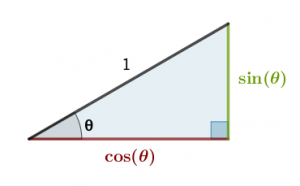For enlargements, the scale factor is the length of the hypotenuse: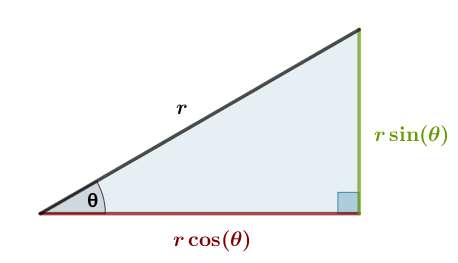# Application

Find the sine and cosine of the angle in the triangle, either using the first applet or a calculator. Multiply by the length of the hypotenuse to find the lengths of the shorter sides. Example of calculation.

# Sines, Cosines and Tangents for any angle

Sine, cosine and tangent have been defined here for angles between 0 and 90 degrees. However, sine, cosine and tangent values can be measured or calculated for any angle. See Visual Trig Values.

Where does the name ‘sine’ come from?